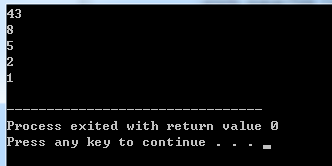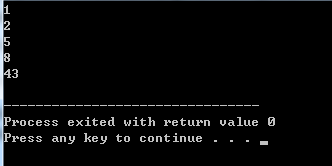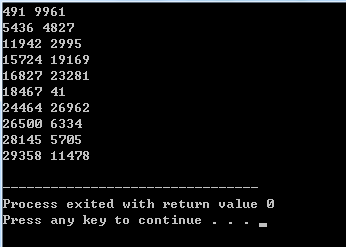2019/04/10 10:10

[TOC]

# C++ cctype头文件内的判断类函数

## （tolower/toupper）实现字母的大小写转换

C:

函数实现原型：
int tolower(int c)//小写
{
if ((c >= 'A') && (c <= 'Z'))
return c + ('a' - 'A');
return c;
}

int toupper(int c)//大写
{
if ((c >= 'a') && (c <= 'z'))
return c + ('A' - 'a');
return c;
}


demo-C语言实现

#include<string.h>   //strlen
#include<stdio.h>    //printf
#include<ctype.h>    //tolower
int main()
{
int i;
char string[] = "THIS IS A STRING";
printf("%s\n", string);
for (i = 0; i < strlen(string); i++)
{
string[i] = tolower(string[i]);
}
printf("%s\n", string);
printf("\n");
}


demo-C++的实现

#include <iostream>
#include <string>
#include <cctype>
using namespace std;
int main()
{
string str= "THIS IS A STRING";
for (int i=0; i <str.size(); i++)
str[i] = tolower(str[i]);
cout<<str<<endl;
return 0;
}


# next_permutation（）

​ #include <algorithm>

​ bool next_permutation(iterator start,iterator end)

#include <iostream>
#include <algorithm>
using namespace std;
int main()
{
int num={1,2,3}; //此时应该是升序，中间出现逆序的会无法出现全部排序
do
{
cout<<num<<" "<<num<<" "<<num<<endl;
}while(next_permutation(num,num+3));
return 0;
}


prev_premutation()与next...相反

# qsort()

void qsort(void *base, size_t nitems, size_t size, int (*compar)(const void *, const void*))


• **base **-- 指向要排序的数组的第一个元素的指针。
• **nitems **-- 由 base 指向的数组中元素的个数。
• **size **-- 数组中每个元素的大小，以字节为单位。
• **compar **-- 用来比较两个元素的函数。

#include <stdio.h>
#include <stdlib.h>

int values[] = { 88, 56, 100, 2, 25 };

int cmpfunc (const void * a, const void * b)
{
return ( *(int*)a - *(int*)b );
}

int main()
{
int n;

printf("排序之前的列表：\n");
for( n = 0 ; n < 5; n++ ) {
printf("%d ", values[n]);
}

qsort(values, 5, sizeof(int), cmpfunc);

printf("\n排序之后的列表：\n");
for( n = 0 ; n < 5; n++ ) {
printf("%d ", values[n]);
}

return(0);
}


# C++ rand 与 srand 的用法

#### rand()

int rand(void)



rand() 的内部实现是用线性同余法做的，它不是真的随机数，因其周期特别长，故在一定的范围里可看成是随机的。

rand() 返回一随机数值的范围在 0 至 RAND_MAX 间。RAND_MAX 的范围最少是在 32767 之间(int)。用 unsigned int 双字节是 65535，四字节是 4294967295 的整数范围。0~RAND_MAX 每个数字被选中的机率是相同的。

rand() 产生的是伪随机数字，每次执行时是相同的; 若要不同, 用函数 srand() 初始化它。

#### srand()

void srand(unsigned int seed)



srand() 用来设置 rand() 产生随机数时的随机数种子。参数 seed 必须是个整数，如果每次 seed 都设相同值，rand() 所产生的随机数值每次就会一样。

### 使用当前时钟作为随机数种子

rand() 产生的随机数在每次运行的时候都是与上一次相同的。若要不同, 用函数 srand() 初始化它。可以利用 srand((unsigned int)(time(NULL)) 的方法，产生不同的随机数种子，因为每一次运行程序的时间是不同的。

### 产生随机数的用法

1. 给srand()提供一个种子，它是一个unsigned int类型；
1. 调用rand()，它会根据提供给srand()的种子值返回一个随机数(在0到RAND_MAX之间)；
1. 根据需要多次调用rand()，从而不间断地得到新的随机数；
1. 无论什么时候，都可以给srand()提供一个新的种子，从而进一步"随机化"rand()的输出结果。

0~RAND_MAX 之间的随机数程序

#include <iostream>
#include <stdlib.h>
#include <time.h>

using namespace std;

int main()
{
srand((unsigned)time(NULL));
for(int i = 0; i < 10;i++ )
cout << rand() << '/t';
cout << endl;
return 0;
}


#### 产生一定范围随机数的通用表示公式

要取得 [a,b) 的随机整数，使用 (rand() % (b-a))+ a;



# stringstream

stringstream 是 C++ 提供的另一个字串型的串流(stream)物件，和之前学过的 iostream、fstream 有类似的操作方式。要使用 stringstream， 必須先加入這一行：

# include <sstream>

stringstream 主要是用在將一個字串分割，可以先用 clear( )以及 str( ) 將指定字串設定成一开始的內容，再用 >> 把个別的资料输出，例如：

string s;
stringstream ss;
int a, b, c;
getline(cin, s);
ss.clear();
ss.str(s);
ss >> a >> b >> c;


3 1 2 3 20 17 23 54 77 60 111 222 333 444 555 666 777 888 999

6 251 4995

string s;
stringstream ss;
int n, i, sum, a;
cin >> n;
getline(cin, s); // 讀取換行
for (i=0; i<n; i++)
{
getline(cin, s);
ss.clear();
ss.str(s);
sum=0;
while (1)
{
ss >> a;
if ( ss.fail() ) break;
sum+=a;
}
cout << sum << endl;
}

					[stringstream使用详解](https://blog.csdn.net/xw20084898/article/details/21939811)


# string substr()函数

string substr (size_t pos = 0, size_t len = npos) const;


string对象。

## 参数

pos 第一个字符的位置被复制为子串。 如果这是等于字符串的长度，该函数返回一个空字符串。 如果这是大于字符串的长度，它会抛出out_of_range。 注意：第一个字符表示为值0（不是1）。 len 字符数在子包括（如果字符串是短，尽可能多的字符可以在需要使用）。 字符串::非营利值表示的所有字符，直到字符串的结尾。

## 返回值

A string object with a substring of this object.

## 实例

#include<string>
using namespace std;
int main()
{
string x="Hello_World";
/*默认截取从0到npos.重载原型为string substr(_off=0,_count=npos);npos一般表示为string类中不存在的位置,_off表示字符串的开始位置，_count截取的字符的数目*/
cout<<x.substr()<<endl;
cout<<x.substr(5)<<endl;//截取x到结尾，即npos.重载原型为string substr(_off,_count=npos)
cout<<x.substr(0,5)<<endl;//以x为始，向后截取5位（包含x），重载原型string substr(_off,_count)
/*
备注：
指定的截取长度加起始位置即_off+_count>源字符串的长度，则子字符串将延续到源字符串的结尾
*/
}


# string append() 函数

## 函数原型

  basic_string &append( const basic_string &str );
basic_string &append( const char *str );
basic_string &append( const basic_string &str, size_type index, size_type len );
basic_string &append( const char *str, size_type num );
basic_string &append( size_type num, char ch );
basic_string &append( input_iterator start, input_iterator end );



## 可实现的功能

append函数常用的三个功能：

• 直接添加另一个完整的字符串:如str1.append(str2);
• 添加另一个字符串的某一段子串:如str1.append(str2, 11, 7);
• 添加几个相同的字符:如str1.append(5, ‘.’);注意,个数在前字符在后.上面的代码意思为在str1后面添加5个"."

## 实例

#include<bits/stdc++.h>
using namespace std;

int main(){
string str1="I like C++";
string str2=",I like the world.";
string str3="Hello";
string str4("Hi");

str1.append(str2);
str3.append(str2, 11, 7);
str4.append(5, '.');

cout<<str1<<endl;
cout<<str3<<endl;
cout<<str4<<endl;
system("pause");
return 0;
}


### 运行结果

I like C++,I like the world.
Hello World.
Hi.....


## substr()和append()结合使用

#### 题目描述

• 连续输入字符串，请按长度为8拆分每个字符串后输出到新的字符串数组；
• 长度不是8整数倍的字符串请在后面补数字0，空字符串不处理。
##### 实现方法1
#include<iostream>
#include<string>
using namespace std;
int main() {
string str;
int count = 0;
int remain_num = 0;
while (cin >> str) {
count = str.size() / 8;
remain_num = str.size() % 8;
for (int i = 0; i < count; i++) {
string full,temp;
for (int j = 0; j < 8; j++) {
temp = str[j];
full += temp;
}
cout << full << endl;
str.erase(str.begin(), str.begin() + 8);
}
if(remain_num){
string remain(8-remain_num, '0');
str += remain;
cout << str << endl;
}
}
}

##### 实现方法2

#include<iostream>
#include<string>
using namespace std;
int main() {
string str;
while (cin >> str) {
int remain_num = str.size() % 8;
while (str.size() >= 8) {
cout << str.substr(0, 8) << endl;
str = str.substr(8);//注意此处代表提取，从str开始到str结尾的内容
}
if (remain_num) {
str.append(8 - remain_num, '0');
cout << str << endl;
}
}
}


# C++11特性的to_string

to_string最常用的就是把int型变量或者数字转化为string类型

double、float也是可以的

#include<iostream>
#include<string>
using namespace std;
int main(){
string s1=to_string(123);
cout<<s1<<endl;
string s2=to_string(4.5);
cout<<s2<<endl;
cout<<s1+s2<<endl;
printf("%s\n",(s1+s2).c_str());//想使用printf输出string的时候要加个.c_str()
}


<br>

# count函数

头文件 algorithm

cout<<count(ivec.begin() , ivec.end() , searchValue)


//读取一系列int数据，并将它们存储到vector对象中，
//然后使用algorithm头文件中定义的名为count的函数，
//统计某个指定的值出现了多少次
#include<iostream>
#include<vector>
#include<algorithm>
using namespace std;

int main()
{
int ival , searchValue;
vector<int> ivec;

//读入int型数据并存储到vector对象中，直至遇到文件结束符
cout<<"Enter some integers(Ctrl+Z to end): "<<endl;
while(cin >> ival)
ivec.push_back(ival);

cin.clear(); // 使输入流重新有效

//读入欲统计其出现次数的int值
cout<<"Enter an integer you want to search: "<<endl;
cin>>searchValue;

//使用count函数统计该值出现的次数并输出结果
cout<<count(ivec.begin() , ivec.end() , searchValue)
<<"  elements in the vector have value "
<<searchValue<<endl;

return 0;
}


## count_if函数

count_if ：返回区间中满足指定条件的元素数目。

template

#include <vector>
#include <algorithm>
#include <iostream>

bool greater10(int value)
{
return value >10;
}

int main()
{
using namespace std;
vector<int> v1;
vector<int>::iterator Iter;

v1.push_back(10);
v1.push_back(20);
v1.push_back(10);
v1.push_back(40);
v1.push_back(10);

cout << "v1 : ";
for (Iter = v1.begin(); Iter != v1.end(); Iter++)
cout << *Iter << " ";
cout << endl;

vector<int>::size_type  result1 = count_if(v1.begin(), v1.end(), greater10);  //count_if算法返回使谓词函数返回条件成立的元素个数
cout << "The number of elements in v1 greater than 10 is: "
<< result1 << "." << endl;

return 0;
}


count_if : 在序列中统计与某谓词匹配的次数

# greater()与less()函数

greater和less是头文件<functional>中定义的两个结构，它们通过重载了()运算符来实现比较功能。

greater的定义如下：

template <class T> struct greater {
bool operator() (const T& x, const T& y) const {return x>y;}
typedef T first_argument_type;
typedef T second_argument_type;
typedef bool result_type;
};


less的定义如下：

template <class T> struct less {
bool operator() (const T& x, const T& y) const {return x<y;}
typedef T first_argument_type;
typedef T second_argument_type;
typedef bool result_type;
};


#include<iostream>
#include<algorithm>//因为用了sort()函数
#include<functional>//因为用了greater<int>()
using namespace std;

void main()
{
int a[]={3,1,4,2,5};
int i;
int len=sizeof(a)/sizeof(int);//这里切记要除以sizeof(int)!

sort(a ,a + len, greater<int>());//内置类型的由大到小排序
for(i=0;i<len;i++)
cout<<a[i]<<" ";
cout<<"\n";
sort(a, a + len, less<int>());//内置类型的由小到大排序
for(i=0;i<len;i++)
cout<<a[i]<<" ";
}


# priority_queue用法

头文件 : #include<queue>



#### 优先输出大数据

priority_queue<Type, Container, Functional>

Type为数据类型， Container为保存数据的容器，Functional为元素比较方式。

#include<iostream>
#include<queue>
using namespace std;

int main(){
priority_queue<int> p;
p.push(1);
p.push(2);
p.push(8);
p.push(5);
p.push(43);
for(int i=0;i<5;i++){
cout<<p.top()<<endl;
p.pop();
}
return 0;
}#### 优先输出小数据

##### 方法一
priority_queue<int, vector<int>, greater<int> > p;


#include<iostream>
#include<queue>
using namespace std;

int main(){
priority_queue<int, vector<int>, greater<int> >p;
p.push(1);
p.push(2);
p.push(8);
p.push(5);
p.push(43);
for(int i=0;i<5;i++){
cout<<p.top()<<endl;
p.pop();
}
return 0;
}##### 方法二：自定义优先级，重载默认的 < 符号

#include<iostream>
#include<queue>
#include<cstdlib>
using namespace std;
struct Node{
int x,y;
Node(int a=0, int b=0):
x(a), y(b) {}
};

struct cmp{
bool operator()(Node a, Node b){
if(a.x == b.x)	return a.y>b.y;
return a.x>b.x;
}
};

int main(){
priority_queue<Node, vector<Node>, cmp>p;

for(int i=0; i<10; ++i)
p.push(Node(rand(), rand()));

while(!p.empty()){
cout<<p.top().x<<' '<<p.top().y<<endl;
p.pop();
}//while
//getchar();
return 0;
}# STL适合场景

vector deque list set mulitset map mulitmap

list 表单 vector 顺序方面元素 deque 队列 map 关键字-值，字典 set 在文本处理过程中，可以用来保存想要忽略的单词。

# isdigit()检查所传的字符是否是十进制数字字符

#### 描述

C 库函数 void isdigit(int c) 检查所传的字符是否是十进制数字字符。

int isdigit(int c);


实例
#include <stdio.h>
#include <ctype.h>

int main()
{
int var1 = 'h';
int var2 = '2';

if( isdigit(var1) )
{
printf("var1 = |%c| 是一个数字\n", var1 );
}
else
{
printf("var1 = |%c| 不是一个数字\n", var1 );
}
if( isdigit(var2) )
{
printf("var2 = |%c| 是一个数字\n", var2 );
}
else
{
printf("var2 = |%c| 不是一个数字\n", var2 );
}

return(0);
}


var1 = |h| 不是一个数字
var2 = |2| 是一个数字


# stoi 函数解析

#### 用法

stoi（字符串，起始位置，n进制），将 n 进制的字符串转化为十进制

stoi(str, 0, 2); //将字符串 str 从 0 位置开始到末尾的 2 进制转换为十进制


#### 头文件

#include <string>


#### 实例

#include <iostream>
#include <string>

using namespace std;

int main()
{
string str = "1010";

int a = stoi(str, 0, 2);

cout << a << endl;
}

//输出10


# iota函数

#### 函数原型

template <class ForwardIterator, class T>
void iota (ForwardIterator first, ForwardIterator last, T val);

template<class _FwdIt,class _Ty> inline
void _Iota(_FwdIt _First, _FwdIt _Last, _Ty _Val)
{   // compute increasing sequence into [_First, _Last)
for (; _First != _Last; ++_First, ++_Val)
*_First = _Val;
}

##### 参数：
• first，last：

分别指向序列中初始及末尾位置的正向迭代器（Forward Iterators）。这个范围即 [first,last) ，包括 first 到 last 间的所有元素，包括 first 指向的元素，但不包括 last 指向的元素。

• val：用于累加的初始值。

#### 实例

// iota example
#include <iostream>     // std::cout
#include <numeric>      // std::iota

int main () {
int numbers;

std::iota (numbers,numbers+10,100);

std::cout << "numbers:";
for (int& i:numbers) std::cout << ' ' << i;
std::cout << '\n';

return 0;
}

numbers: 100 101 102 103 104 105 106 107 108 109### 作者的其它热门文章

0
0 收藏

0 评论
0 收藏
0